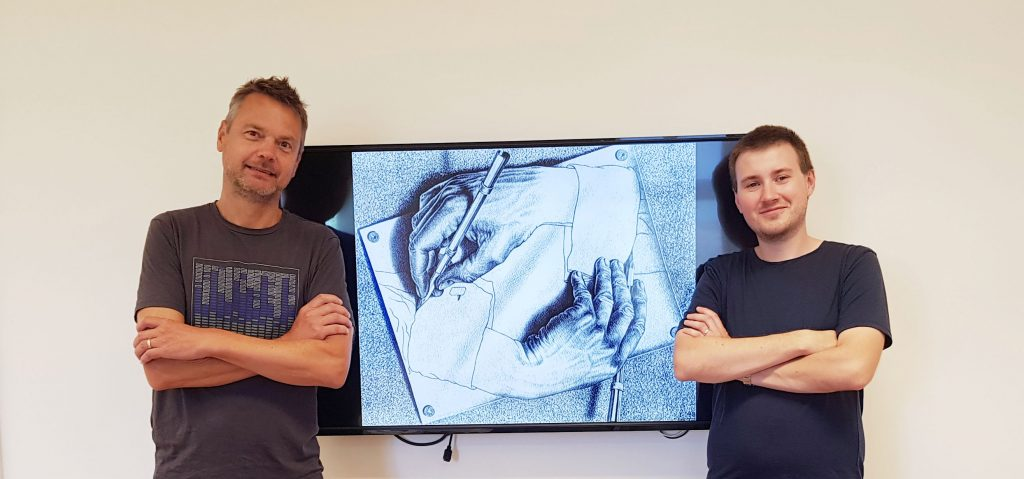### Best Paper Award at CP’2020

Tomáš Peitl and Stefan Szeider won the Best Paper Award at the main track of CP'2020, the 26th International Conference on Principles and Practice of Constraint Programming, for their paper: "Finding the Hardest Formulas for Resolution".

Congratulations!

In the paper, a  resolution-based method (CDCL SAT solver) is used to find the hardest formulas for resolution, which constitutes a self reference as greatly illustrated by MC Escher in his lithograph "Drawing Hands (1948).Abstract: A CNF formula is harder than another CNF formula with the same number of clauses if it requires a longer resolution proof. The resolution hardness numbers give for 𝑚=1,2,…m=1,2,… the length of a shortest proof of a hardest formula on m clauses. We compute the first ten resolution hardness numbers, along with the corresponding hardest formulas. We achieve this by a candidate filtering and symmetry breaking search scheme for limiting the number of potential candidates for formulas and an efficient SAT encoding for computing a shortest resolution proof of a given candidate formula.

An open access version of the paper is available as a technical report